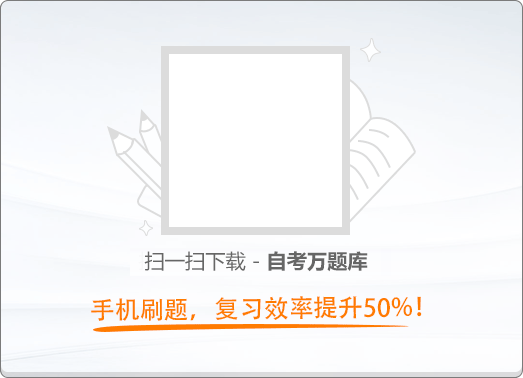150:002017年4月《大学语文》真题
（本大题共20小题，每小题1分，共20分。在每小题列出的四个备选项中只有一个是符合题目要求的，请将其代码填写在题后的括号内。错选、多选或未选均无分。）
1
(单项选择题)

• A.

礨空之在大泽

• B.

中国之在海内

• C.

稊米之在大仓

• D.

毫末之在于马体

• A
• B
• C
• D

2
(单项选择题)

• A.

顺与逆

• B.

成与败

• C.

强与弱

• D.

幸与蹇

• A
• B
• C
• D

3
(单项选择题)

《吃饭》提及的古人中，被作者认为“早已明白”“音乐的道理可通于烹饪”的是（　）。

• A.

伊尹

• B.

老子

• C.

孔子

• D.

孟子

• A
• B
• C
• D

4
(单项选择题)

《冯谖客孟尝君》中，“冯谖不复歌”的根本原因是（　）。

• A.

出有车

• B.

孟尝君左右的厌恶

• C.

食有鱼

• D.

满意孟尝君的宽容

• A
• B
• C
• D

5
(单项选择题)

• A.

背诵《汉书》

• B.

记百姓名姓

• C.

拔刀断一指

• D.

操纸笔立书

• A
• B
• C
• D

6
(单项选择题)

《赵武灵王胡服骑射》中，赵武灵王推行胡服，而“国人皆不欲”的原因是（　）。

• A.

胡服过于窄小

• B.

公子成极力反对

• C.

胡服便于骑射

• D.

夷夏有别的观念

• A
• B
• C
• D

7
(单项选择题)

《前赤壁赋》中描写洞箫的语句是（　）。

• A.

纵一苇之所如，凌万顷之茫然

• B.

挟飞仙以遨游，抱明月而长终

• C.

舞幽壑之潜蛟，泣孤舟之嫠妇

• D.

耳得之而为声，目遇之而成色

• A
• B
• C
• D

8
(单项选择题)

• A.

楼船箫鼓，峨冠盛筵，灯火优傒

• B.

杭人游湖，巳出酉归，避月如仇

• C.

岸上人亦逐队赶门，渐稀渐薄

• D.

吾辈纵舟，酣睡于十里荷花之中

• A
• B
• C
• D

9
(单项选择题)

《香市》中说“从前农村还是'桃源'的时候”作者是想说明（　）。

• A.

从前的农村有着最理想的经济形态

• B.

大革命破坏了农村原有的自然风光

• C.

往日农村尽管人迹罕至，但香市热闹非凡

• D.

农村自然经济被破坏，新变化未带来繁荣

• A
• B
• C
• D

10
(单项选择题)

《爱尔克的灯光》中故居照壁上“长宜子孙”四个字引发的作者的思想感情是（　）。

• A.

对遥远旧梦的怀念

• B.

对姐姐不幸遭遇的痛惜

• C.

对祖辈的亲密回忆

• D.

对封建家庭的彻底否定

• A
• B
• C
• D

11
(单项选择题)

《纪念傅雷》所叙述的事例中，能说明傅雷“在青年时候，他的刚直还近于狂妄”的是（　）。

• A.

与滕固吵翻，一怒返沪

• B.

成为第五类分子

• C.

望子成龙，逼傅聪练琴

• D.

不满“墨猪”之评

• A
• B
• C
• D

12
(单项选择题)

• A.

小弟说起这话时仍然面带笑容，毫不介意

• B.

他站着上课，那神色真是庄严

• C.

小弟白面长身，美丰仪；喜文艺，娴诗词

• D.

他站在山泉边，捧着一个大盆的样子，至今还十分清晰地在我眼前

• A
• B
• C
• D

13
(单项选择题)

• A.

忘言

• B.

人境

• C.

南山

• D.

心远

• A
• B
• C
• D

14
(单项选择题)

• A.

蜀江水碧蜀山青，圣主朝朝暮暮情

• B.

芙蓉如面柳如眉，对此如何不泪垂

• C.

楼阁玲珑五云起，其中绰约多仙子

• D.

七月七日长生殿，夜半无人私语时

• A
• B
• C
• D

15
(单项选择题)

《鹧鸪天》（重过阊门万事非）使用反诘句“同来何事不同归”、“谁复挑灯夜补衣”所达到的抒情效果是（　）。

• A.

重章叠句，创造凄美的意境，意在言外

• B.

突兀而起，把情感推向高潮，动人心弦

• C.

凸显事理颠倒的假象，表达内心的焦虑不安

• D.

增强语气，表达屈居下僚、未尽其才的愤懑

• A
• B
• C
• D

16
(单项选择题)

• A.

叹年来踪迹，何事苦淹留

• B.

乍暖还寒时候，最难将息

• C.

雁过也，正伤心，却是旧时相识

• D.

算只有殷勤，画檐蛛网，尽日惹飞絮

• A
• B
• C
• D

17
(单项选择题)

《摸鱼儿》（更能消几番风雨）：“君莫舞，君不见、玉环飞燕皆尘土！”其中“君莫舞”二字的深层情感内涵是（　）。

• A.

警告谗害忠良者

• B.

感慨历史兴亡

• C.

惋惜春天的离去

• D.

讽刺红颜祸水

• A
• B
• C
• D

18
(单项选择题)

《祖国啊，我亲爱的祖国》“把纤绳深深/勒进你的肩膊，一一祖国呵”的象征意义是（　）。

• A.

饱受压迫的下层劳动人民的苦难生活

• B.

经历文革动乱的一代青年的痛苦记忆

• C.

贫穷落后的历史面貌给予祖国的沉重负载

• D.

源远流长的历史传统赋予青年的重大责任

• A
• B
• C
• D

19
(单项选择题)

《婴宁》中婴宁的自然天性受到社会束缚的具体表现是（　）。

• A.

矢不复笑

• B.

忍笑而立

• C.

笑辄不辍

• D.

反笑为哭

• A
• B
• C
• D

20
(单项选择题)

• A.

《断魂枪》

• B.

《金鲤鱼的百裥裙》

• C.

《苦恼》

• D.

《麦琪的礼物》

• A
• B
• C
• D